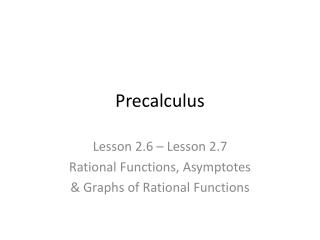Download PresentationPrecalculus

# Precalculus - PowerPoint PPT PresentationDownload Presentation## Precalculus

- - - - - - - - - - - - - - - - - - - - - - - - - - - E N D - - - - - - - - - - - - - - - - - - - - - - - - - - -
##### Presentation Transcript

1. Precalculus Lesson 2.6 – Lesson 2.7 Rational Functions, Asymptotes & Graphs of Rational Functions

2. Find the domain of the rational functions

3. Vertical Asymptotes If the rational function is in lowest terms (no common factors of N and D), then: The graph of f has vertical asymptotes at the zeros of D(x).

4. x = c y x x = c y x

5. Find the vertical asymptotes, if any, of the graph of the rational functions:

6. 1. If n < m, then the line y = 0 (x-axis) is a horizontal asymptote of the graph of f. 2. If n = m, then the line y = an / bmis a horizontal asymptote of the graph of f. 3. If n = m + 1, then y = ax + b is a slant (oblique) asymptote of the graph of f. Found using long division. 4. If n > m + 1, the graph of f has neither a horizontal nor a slant (oblique) asymptote.

7. y y = f(x) y = L x y y = L x y = f(x)

8. If an asymptote is neither horizontal nor vertical it is called oblique. y x

9. Find the horizontal asymptotes of the graph of the rational function, if any:

10. Point discontinuity is like a hole in a graph. If the original related expression is undefined for x a but the simplified expression is defined for x a, then there is a hole in the graph at x a.

11. Analytical and Numerical Explanations • Determine the domains of f and g. • Simplify f and find any vertical asymptotes. • Complete the table. • Explain how the two functions differ.

12. Plotting data on your graphing calculator:

13. To Enter Data in a List: • Press the STAT button. • Press 1 to edit. • L1 will be your independent variable (x). • L2 will be your dependent variable (y).

14. The information that you entered into your lists represents the number N of threatened and endangered species in the United States from 1993-2002. The data can be approximated using the equation: t represents the year, with t = 3 corresponding to 1993 • Use your graphing calculator to plot the data and graph the function in the same viewing window. How closely does the function represent the data? • Use the function to estimate the number of threatened and endangered species in 2006. • Would this model be useful for estimating the number of threatened and endangered species in future years? Explain?

15. Graphing Rational Functions • Simplify the function if possible. • Find and plot the y-intercept (if any) by evaluating f(0). • Find the zeros of the numerator by solving the equation N(x) = 0. Then plot the corresponding x-intercepts. • Find the zeros of the denominator by solving the equation D(x) = 0. Then sketch the corresponding vertical asymptotes using dashed vertical lines. • Find and sketch the horizontal asymptotes (if any) using dashed horizontal lines. • Find and sketch any slant asymptotes (if any) using a dashed line. • Find and plot any holes. • Plot at least one point between and one point beyond each x-intercept and vertical asymptote. • Use smooth curves to draw your graph.

16. Sketch the Graph by Hand:

17. Sketch the Graph by Hand:

18. Sketch the Graph by Hand:

19. Sketch the Graph by Hand:

20. Sketch the Graph by Hand:

21. Application: Finding a Minimum Area (Calculus Preview) A rectangular page is designed to contain 48 square inches of print. The margins on each side of the page are 1.5” wide. The margins at the top and bottom are each 1” deep. What should the dimensions of the page be so that the minimum amount of paper is used?